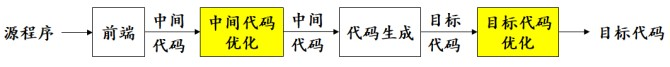• 掌握基本块优化技术，主要包括：
• 公共表达式的删除、复制传播、常数合并及常数传播、削弱计算强度等；
• 掌握循环优化技术，主要包括：
• 循环展开、代码外提、削弱计算强度、删除归纳变量；

## 概述

• 对中间代码或目标代码进行等价变换，使变换后的代码质量更高。

• 等价变换
• 提高目标代码的执行速度
• 减少目标代码占用的空间

• 目标代码生成之前的中间代码优化
• 目标代码生成之后的目标代码优化## 基本块优化

i = 0
--------------
LOOP: i = i + 1 // 不能优化为 i = 1
...
if i < n goto LOOP

• 常数合并
• 计算常数表达式。$x= 3*2+1 \Rightarrow x = 7$
• 常数传播
• 常量作为值代入表达式
• 删除公共表达式
• 反复使用某个求值，而求值所依赖的变量状态皆未改变，则没必要反复求值，用第一次的即可。
• 复制传播
• a = b, c = a，则后文实际上都可以用 b 来表示 a, c
• 删除死代码
• 求值后却没有使用的值
• 无法到达的块（如恒假条件里的块）
• 死块的后面很可能也是死块
• 削弱计算强度
• 例如 x = y**2 变成 x = y * y
• 例如 x = x + 0, x = 1 * x 可能是无意义的。
• 改变计算次序（没看懂有何意义）

## 循环优化

• 初始化部分
• 测试部分
• 循环体
• 调节部分 i++

• 循环展开：针对固定次数循环。以空间换时间。
• 代码外提 / 频度削弱：将循环结构中的循环无关代码提到循环结构的外面。
• 削弱计算强度
• 删除归纳变量：对于一个变量 $x$ ，每次 $x$ 被赋值都只是简单地增加常数 $c$，则 $x$ 是一个归纳变量。可以把循环计算变成简单的增量运算。
int k;
for(unsigned i = 0; i < n; ++i) {
k = k + 3;
// ... else
}


int k = 3 * n;
for(unsigned i = 0; i < n; ++i) {
// ... else
}